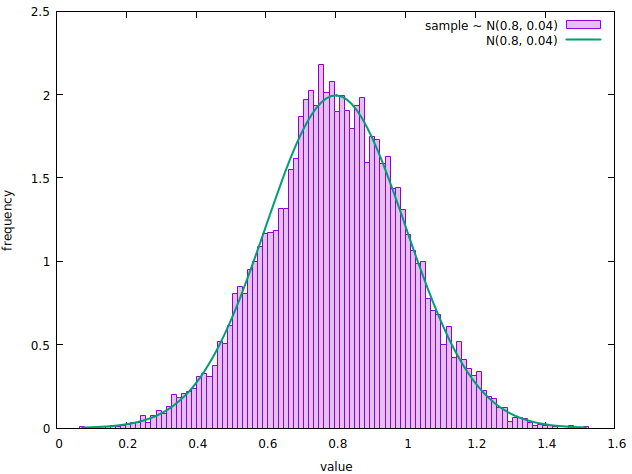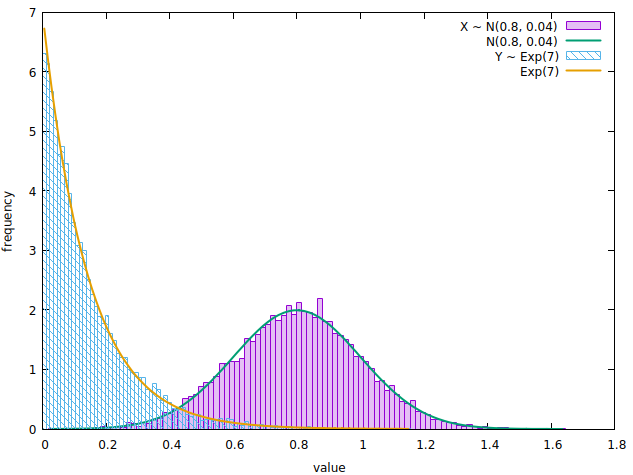{{ message }}

Instantly share code, notes, and snippets.

# lbarasti/normal_distribution.cr

Last active May 4, 2020
Sampling random variables and plotting histograms in Crystal

This is a companion gist to the article Sampling random variables and plotting histograms in Crystal

## Prerequisite

• an installation of crystal `0.33.0` or higher.
• an installation of Gnuplot

## How to run

Clone the files in this gist, then run the following once, to install the code dependencies.

``````shards install
``````

You can now plot some histograms with

``````crystal normal_distribution.cr
``````

and

``````crystal normal_vs_exp.cr
``````
 require "statistics" require "ishi" require "tablo" include Statistics::Distributions # Turns a named tuple into tabular representation def table(data : NamedTuple) Tablo::Table.new(data.map { |k, v| [k, v] }, header_frequency: nil) { |t| t.add_column("coefficient") { |n| n } t.add_column("value") { |n| n.as(Float64).round(3) } } end normal = Normal.new(mean: 0.8, std: 0.2) size = 10000 sample = (0...size).map { normal.rand } info = Statistics.describe(sample) puts table(info) bins = 100 sample_bins = Statistics.bin_count(sample, bins, edge: :centre) x = sample_bins.edges y = sample_bins.counts area = size * sample_bins.step normalized_y = y.map &./(area) # Evaluate the probability density function in `x` pdf = x.map { |x| normal.pdf(x) } Ishi.new do plot(x, normalized_y, title: "sample \\~ N(0.8, 0.04)", style: :boxes, fs: 0.25) .xlabel("value") .ylabel("frequency") plot(x, pdf, title: "N(0.8, 0.04)", lw: 2, ps: 0) end
 require "statistics" require "ishi" include Statistics::Distributions normal = Normal.new(mean: 0.8, std: 0.2) expo = Exponential.new(lambda: 7) size = 10000 sample_1 = (0...size).map { normal.rand } sample_2 = (0...size).map { expo.rand } bins = 100 sample_1_bins = Statistics.bin_count(sample_1, bins, edge: :centre) sample_2_bins = Statistics.bin_count(sample_2, bins, edge: :centre) x_1 = sample_1_bins.edges area_1 = size * sample_1_bins.step y_1 = sample_1_bins.counts.map &./(area_1) normal_pdf = x_1.map { |x| normal.pdf(x) } x_2 = sample_2_bins.edges area_2 = size * sample_2_bins.step y_2 = sample_2_bins.counts.map &./(area_2) expo_pdf = x_2.map{|i| expo.pdf(i)} Ishi.new do plot(x_1, y_1, title: "X \\~ N(0.8, 0.04)", style: :boxes, fs: 0.25) plot(x_1, normal_pdf, title: "N(0.8, 0.04)", lw: 2, ps: 0) plot(x_2, y_2, title: "Y \\~ Exp(7)", style: :boxes, fs: 4) plot(x_2, expo_pdf, title: "Exp(7)", lw: 2, ps: 0) .xlabel("value") .ylabel("frequency") end
 name: sampling_and_plotting version: 0.1.0 authors: - lbarasti dependencies: ishi: github: toddsundsted/ishi branch: master statistics: github: lbarasti/statistics branch: master tablo: github: hutou/tablo version: 0.9.3 crystal: 0.34.0 license: MIT

###lbarasti commented Apr 16, 2020

 Output of `normal_distribution.cr`###lbarasti commented Apr 16, 2020

 Output of `normal_vs_exp.cr`